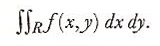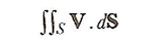A# surface integral

The surface integral is the double integral obtained through integration of a function f = (x, y) over a surface S whose vertical projection on the xy-plane in the region of R. The surface integral gives the volume between the surface and the xy-plane and is writtenIn vector notation (see vector analysis), the surface integral is generally writtenwhich is a scalar, dS being a surface element treated as the vector dx × dy and V being a vector function.